# Python for循环嵌套

break是终止循环、else后面的代码hi在循环正常结束并且不被打断情况下执行、continue是立即终止本次循环，进入下一次循环。

``````username = '110119'
num = 0
while num < 3:
print("登入成功")
while True:
action = input('请输入你的操作')
if action == 'xxx':
break
print(f'正在看{action}')
break
else:
print('用户名或密码错误，请注意格式大小写')
num += 1
else:
print('账号密码输错三次，已被锁定')````````````username = '110119'
num = 0
for i in range(3):
print("登入成功")
while True:
action = input('请输入你的操作')
if action == 'q':
break
print(f'正在看{action}')
break
else:
print('用户名或密码错误，请注意格式大小写')
num += 1
else:
print('账号密码输错三次，已被锁定')``````

break、else、continue这三个效果都是一样的。直接运行，输错三次账号密码，就退出了循环，然后账号密码被锁定。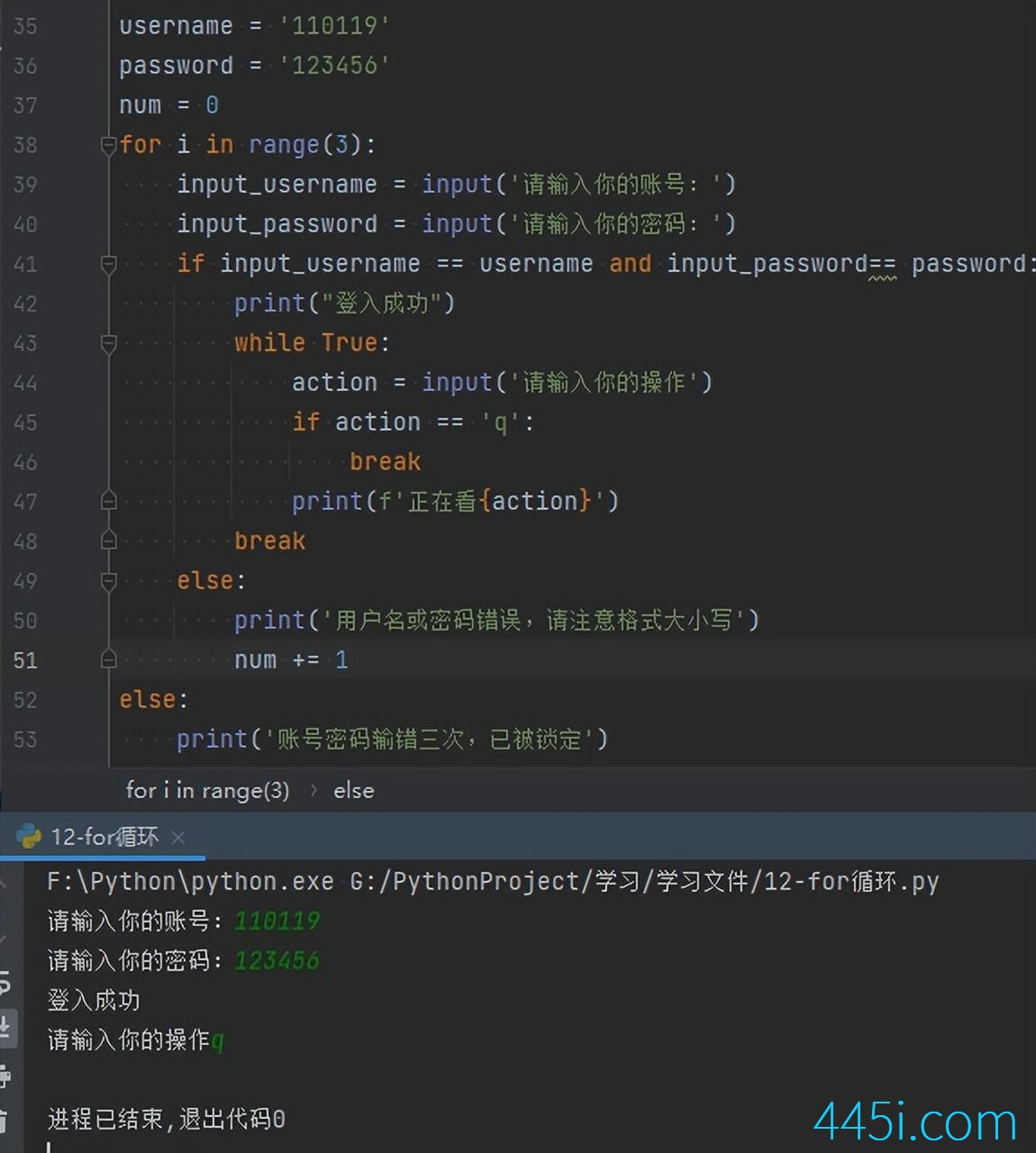``````or i in range(1,10):
print('外层循环---->',i)``````

``````for i in range(1,10):
print('外层循环---->',i)
for x in range(1, 10):
print('内层循环-->', x)``````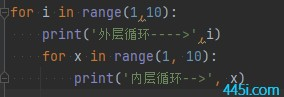——

——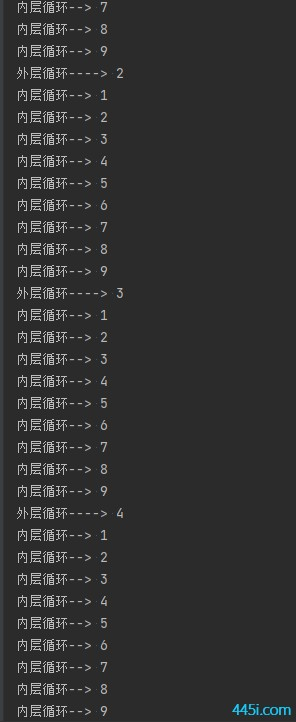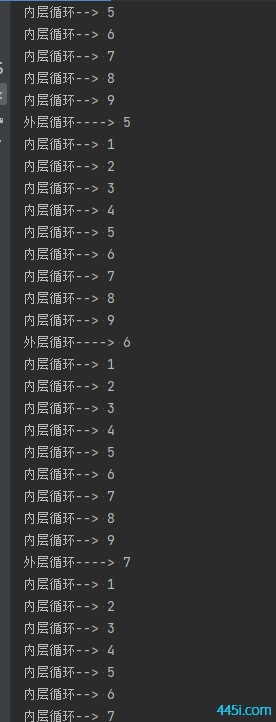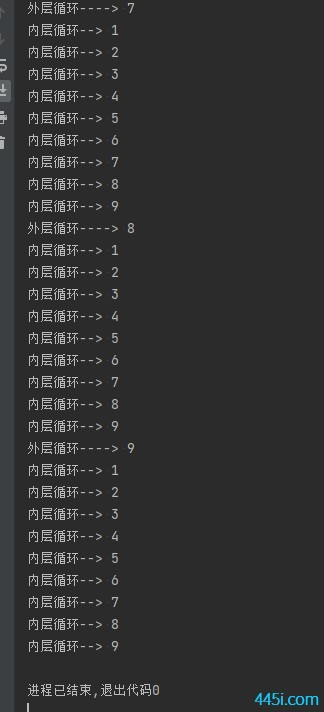### 觉得文章有用就打赏一下文章作者

#### 支付宝扫一扫打赏#### 微信扫一扫打赏